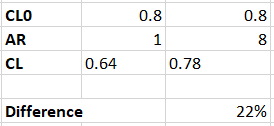# High vs low ratio

I am doing an experiment in school were I will build two different foil wings and then test their lift in water. The idea is that I will be testing one high aspect wing and one low aspect wing. However I am not sure of the messurements, do you have any idea about how big the aspect difference should be? Or how the wings should look to get the best results? (The purpose is the get different results with the different wings so it does not matter if one of them preforms better)

1 Like

will these 2 wings have the same surface area?

Yes. I think that makes it easier to compare.

I am not expert in wing but I will use same area with a ratio of 2 to 3 ( between low and high ) if that was the question …
Usually aspect goes from 3,5 to 9 …

Just a suggestion, you can use this online wing design software to play with different parameters and see how it affect the aspect ratio with the wing metrics option enabled:

Hi Olivia

Sounds like an interesting experiment.

Here is a reference for the effect of aspect ratio on the coefficient of lift, it also provides some explanation.
https://www.grc.nasa.gov/www/k-12/airplane/downwash.html

If we take a NACA 2412 airfoil at 5 degree angle of attack this gives a coefficient of lift of ~0.8. An then if we take a AR of 2 and 8, we can see this would generate a difference in lift of 22%, which would hopefully be measurable.The equation of lift is as follows:
L = C_L / 2 * A * rho * v^2
Where

• L is lift
• C_L is the coefficient of lift
• A is the planform area
• rho is the density
• v is the velocity

So for your experiment to be successful you’ll need to ensure

1. You can accurately measure lift
2. You control the other variables such as planform area and velocity

What is your experimental setup out of interest? And how do you plan to measure the lift force?